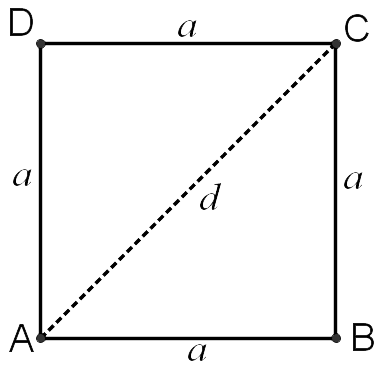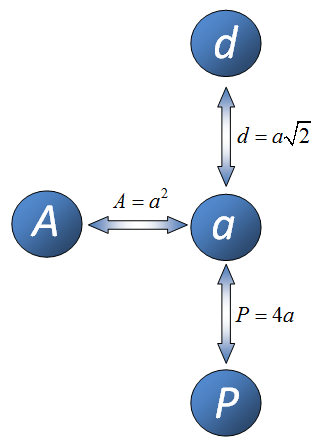Math Calculators, Lessons and Formulas

It is time to solve your math problem

mathportal.org

# Square calculator

Enter one known value and calculate the side, area, perimeter or diagonal of a square. You will get solution with step-by-step explanation.

Square Calculator
Input value you know and select what to compute.( show help ↓↓ )
0123456789/.del
I want to calculate:
view examples ↓↓
Input one element of a square
use letter r to input square root. Ex: $3\sqrt{2} = \text{3r2}$
side $a$
=area $A$
=
perimeter $P$
=
diagonal $d$
=

examples
example 1:ex 1:
Find the area of a square of diagonal $d = 12 cm$.
example 2:ex 2:
Find the side of the square whose perimeter is $d = 14 cm$.
example 3:ex 3:
Find the diagonal of the square whose area is $A = 18 cm^2$.

# Formulas for Square

To calculate missing value in square, based on one known value, you need to remember just three formulas.F1: $$P = 4 \cdot a$$ a = side d = diagonal A = area P = perimeter F2: $$A = a^2$$ F3: $$d = a \cdot \sqrt{2}$$

The diagram at the right shows when to use each of these formulas.

## Example 1:

If you are given diagonal d and you want to calculate side a, then you need to use formula which connects d and a.

## Example 2:

If you are given area A and you want to calculate perimeter P then you need to make two steps to calculate perimeter. In first step you apply formula which connects area A and side a after that use formula which connects side a and perimeter P.

Quick Calculator Search